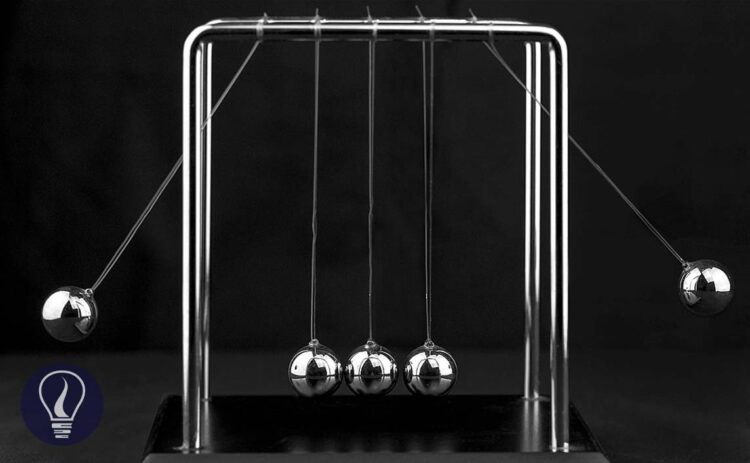More results...

Generic selectors
Exact matches only
Search in title
Search in content
Post Type Selectors

# Linear MomentumB.Sc (Hons, USJ) (Polymer Science and Technology, Chemistry, Physics)
Categories
Last Updated On : August 30, 2023
Published Date : August 30, 2023

Momentum is the ability to do work. A moving object has momentum due to the velocity of the object and the mass of the object. Anything that has a mass and a velocity has momentum. The motion can be linear or angular motion.

When the object is moving on a linear path it has a linear momentum. When the object is in an angular motion, it has an angular momentum.

## Linear momentum of an object

The linear momentum of an object is given by the product of its mass and velocity.

Where,

• P = Linear momentum
• m = Mass of the object
• V = Velocity of the object

Mass is a scalar. But the velocity is a vector. Therefore, linear momentum is a vector too. That means linear momentum has a direction. The direction of the momentum is the same direction of the velocity.

Question 01

What is the momentum of an object with 200g of mass, when it is moving with 400 ms-1 velocity?

Solution

Question 02

A liquid of density 1000 kgm-3 is ejected with a velocity of 4 ms-1 from a tube of cross-sectional area 0.25 m2 What is the momentum of the flowing liquid in one second?

Solution

Velocity is the distance traveled at 1s. Therefore, the distance traveled by the liquid in 1 second is 4 m.

## Rate of change of momentum

When a moving object changes its velocity, the momentum of the object also changes. The rate of change of momentum is the change of the momentum of an object with respect to time.

If an object changes its velocity by ΔV and its momentum has changed by ΔΡ at a time "t", we can write the momentum equation for the object as follows,

But ΔV/t is the rate of change of velocity. That means the acceleration (or de-acceleration) of the object. Therefore, the rate of change of the momentum equals the product of mass and the acceleration of the object.

From Newton's second law, the rate of change of the momentum is the force (F) that acts on the object. Therefore, we can re-write the equation as follows

Question 03

An object with 4 kg of mass changes its velocity from 20 ms-1 to 100 ms-1 within 2 seconds. Find the rate of change of momentum of the object.

Solution

Question 04

A bouncing ball with 200 g of mass vertically hits the ground with 4 ms-1 velocity. The ball bounces back vertically upwards with the same velocity after it hits the ground. Find the change in the momentum of the ball.

Solution

Here, even the ball does not change the magnitude of the velocity, it changes the direction of the velocity. If we assume that the vertically downward direction is the positive direction, the vertically upward direction is the negative direction.

Therefore, the velocity when the ball moves towards the ground is +4 ms-1, and when the bounces back, the velocity is -4 ms-1.

Here the negative sign indicates that the change of the momentum is in the negative direction.

## Conservation of momentum

Until an external force is applied, the momentum of two or more bodies that are acting upon each other in an isolated system is a constant. The total initial momentum is equal to the total final momentum. Therefore, the momentum cannot be created or destroyed.

Let's consider a situation where two balls of A and B move towards each other and collide. After the collision, two balls will bounce back and move the same initial way but in the opposite direction.

The two balls of A and B have masses of m1 and m2 respectively. The initial velocities of A and B are U1 and U2, after the collision they have V1 and V2 velocities respectively.

As momentum is a vector, we should also consider the direction when we apply the law of conservation of momentum. In the above situation, if we take ball A is moving in the positive direction and ball B is moving negative direction, we can re-write the above equation as follows.

Question 05

An object with 60 kg of mass suddenly explodes into two parts. One part is 40 kg and it moves on east with 25 ms-1 and the other part is 20 kg and it moves to the west. What is the velocity of the 20kg piece?

Solution

At the initial state, the object is at rest. That means there is no velocity and therefore, the initial momentum is zero. Let's take the velocity of a 20 kg piece as V.## References and Attributes

### Figures:

The cover image was created using an image by Sheila Sund from Salem, United States, licensed under CC BY 2.0, via Wikimedia Commons